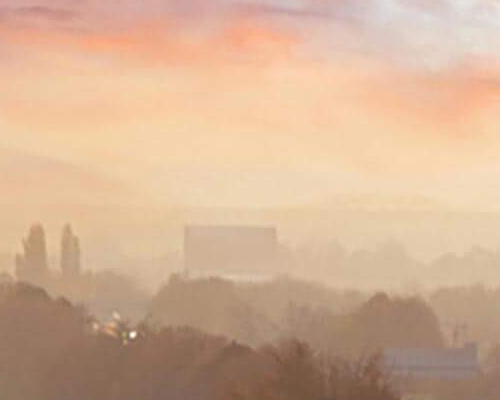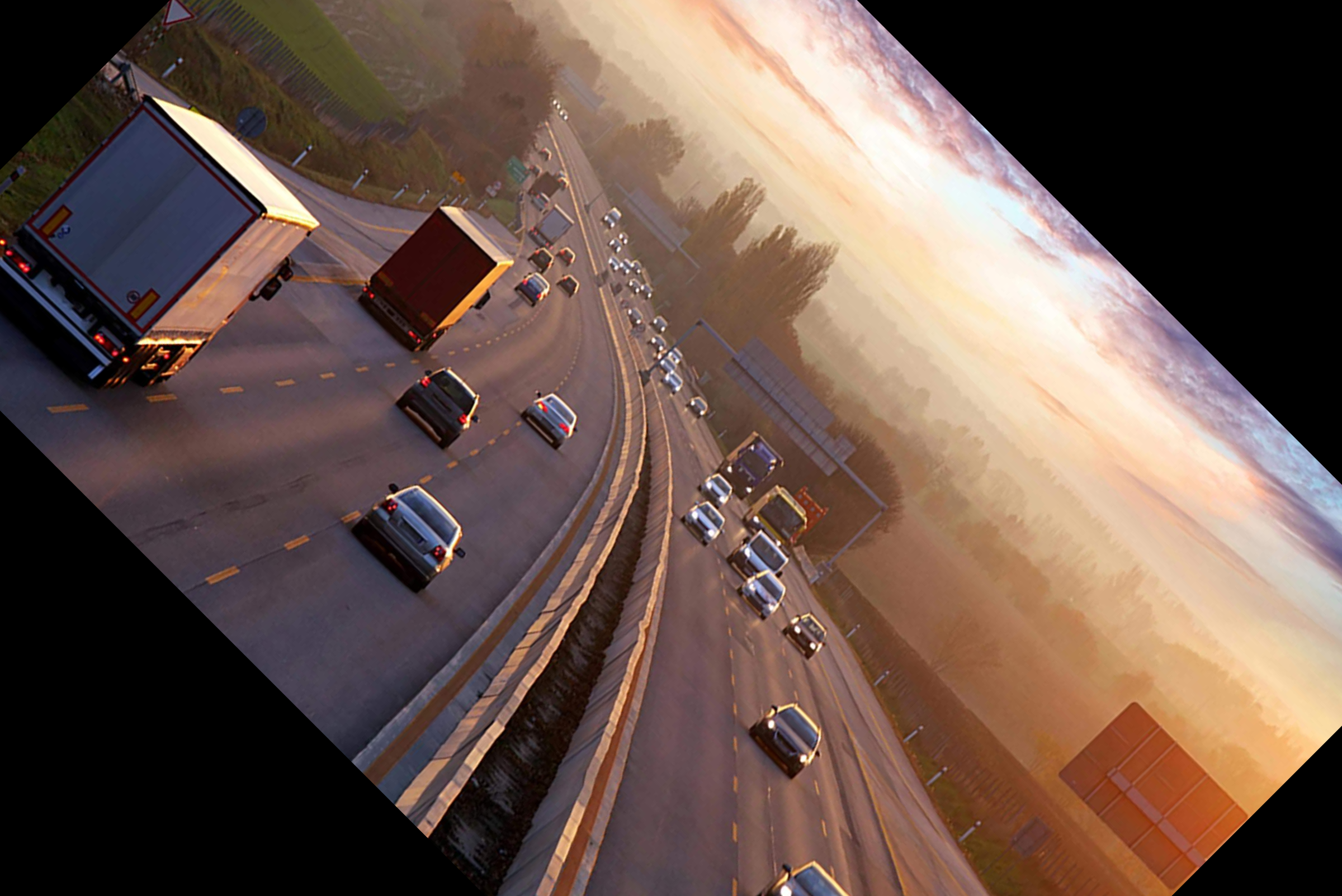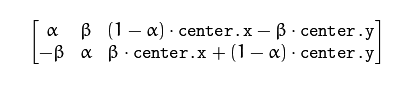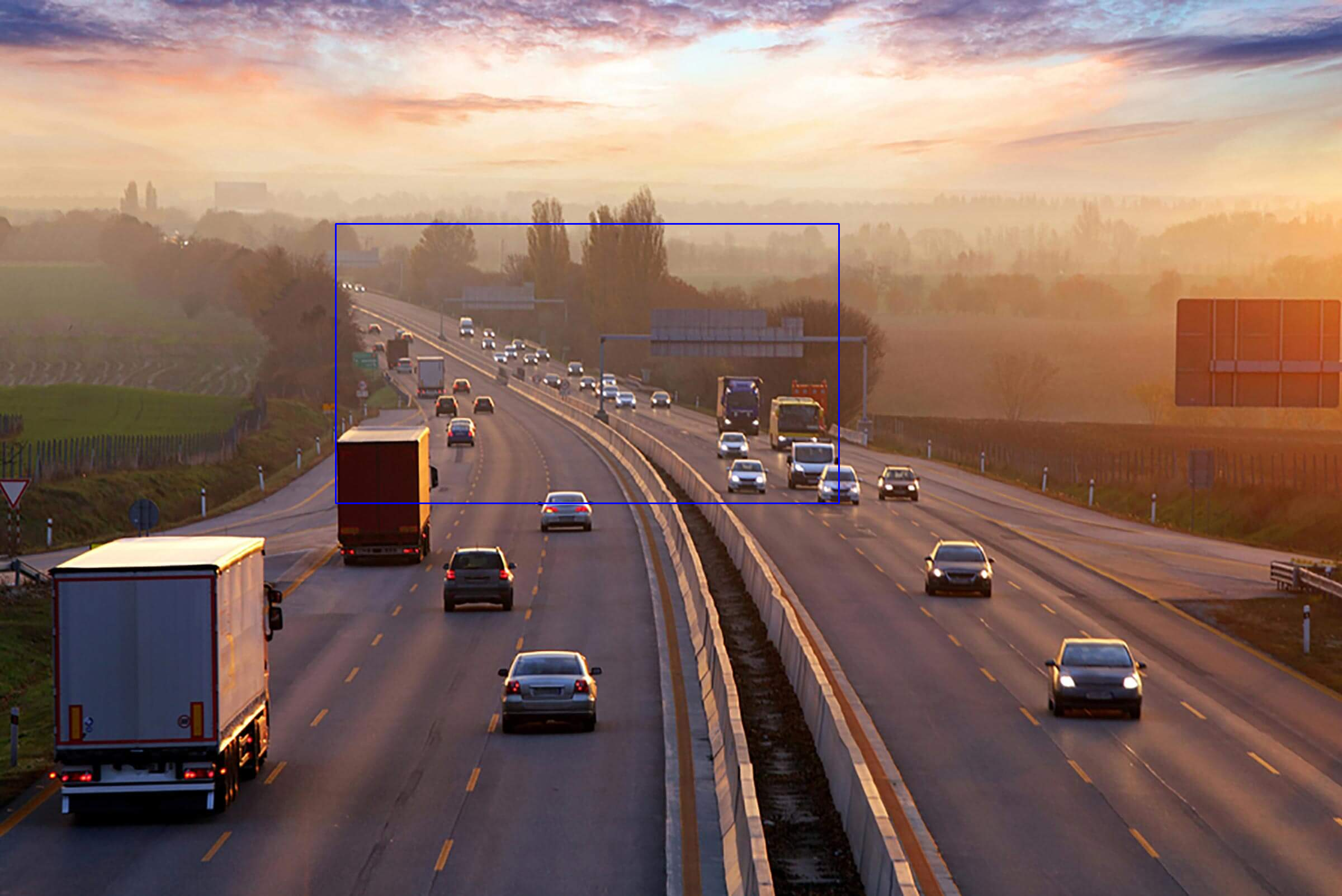# Introduction to OpenCV

OpenCV is one of the most popular computer vision libraries. If you want to start your journey in the field of computer vision, then a thorough understanding of the concepts of OpenCV is of paramount importance.
In this article, I will try to introduce the most basic and important concepts of OpenCV in an intuitive manner.

2. Extracting the RGB values of a pixel
3. Extracting the Region of Interest (ROI)
4. Resizing the Image
5. Rotating the Image
6. Drawing a Rectangle
7. Displaying text

This is the original image that we will manipulate throughout the course of this article.`# Importing the OpenCV library ` `import` `cv2 ` `# Reading the image using imread() function ` `image ``=` `cv2.imread(``'image.png'``) ` ` `  `# Extracting the height and width of an image ` `h, w ``=` `image.shape[:``2``] ` `# Displaying the height and width ` `print``(``"Height = {},  Width = {}"``.``format``(h, w)) `

Now we will focus on extracting the RGB values of an individual pixel.
Note – OpenCV arranges the channels in BGR order. So the 0th value will correspond to Blue pixel and not Red.

Extracting the RGB values of a pixel

 `# Extracting RGB values.  ` `# Here we have randomly chosen a pixel ` `# by passing in 100, 100 for height and width. ` `(B, G, R) ``=` `image[``100``, ``100``] ` ` `  `# Displaying the pixel values ` `print``(``"R = {}, G = {}, B = {}"``.``format``(R, G, B)) ` ` `  `# We can also pass the channel to extract  ` `# the value for a specific channel ` `B ``=` `image[``100``, ``100``, ``0``] ` `print``(``"B = {}"``.``format``(B)) `

Extracting the Region of Interest (ROI)

 `# We will calculate the region of interest  ` `# by slicing the pixels of the image ` `roi ``=` `image[``100` `: ``500``, ``200` `: ``700``] `Resizing the Image

 `# resize() function takes 2 parameters,  ` `# the image and the dimensions ` `resize ``=` `cv2.resize(image, (``800``, ``800``)) `The problem with this approach is that the aspect ratio of the image is not maintained. So we need to do some extra work in order to maintain a proper aspect ratio.

 `# Calculating the ratio ` `ratio ``=` `800` `/` `w ` ` `  `# Creating a tuple containing width and height ` `dim ``=` `(``800``, ``int``(h ``*` `ratio)) ` ` `  `# Resizing the image ` `resize_aspect ``=` `cv2.resize(image, dim) `Rotating the Image

 `# Calculating the center of the image ` `center ``=` `(w ``/``/` `2``, h ``/``/` `2``) ` ` `  `# Generating a rotation matrix ` `matrix ``=` `cv2.getRotationMatrix2D(center, ``-``45``, ``1.0``)  ` ` `  `# Performing the affine transformation ` `rotated ``=` `cv2.warpAffine(image, matrix, (w, h)) `There are a lot of steps involved in rotating an image. So, let me explain each of them in detail.

The 2 main functions used here are –

• getRotationMatrix2D()
• warpAffine()

getRotationMatrix2D()
It takes 3 arguments –

• center – The center coordinates of the image
• Angle – The angle (in degrees) by which the image should be rotated
• Scale – The scaling factor
•
It returns a 2*3 matrix consisting of values derived from alpha and beta
alpha = scale * cos(angle)
beta = scale * sine(angle)warpAffine()

The function warpAffine transforms the source image using the rotation matrix:

```dst(x, y) = src(M11X + M12Y + M13, M21X + M22Y + M23)
```

Here M is the rotation matrix, described above.
It calculates new x, y co-ordinates of the image and transforms it.

Drawing a Rectangle
It is an in-place operation.

 `# We are copying the original image,  ` `# as it is an in-place operation. ` `output ``=` `image.copy() ` ` `  `# Using the rectangle() function to create a rectangle. ` `rectangle ``=` `cv2.rectangle(output, (``1500``, ``900``),  ` `                          ``(``600``, ``400``), (``255``, ``0``, ``0``), ``2``) `It takes in 5 arguments –

• Image
• Top-left corner co-ordinates
• Bottom-right corner co-ordinates
• Color (in BGR format)
• Line width

Displaying text
It is also an in-place operation

 `# Copying the original image ` `output ``=` `image.copy() ` ` `  `# Adding the text using putText() function ` `text ``=` `cv2.putText(output, ``'OpenCV Demo'``, (``500``, ``550``),  ` `                   ``cv2.FONT_HERSHEY_SIMPLEX, ``4``, (``255``, ``0``, ``0``), ``2``) `It takes in 7 arguments –

1. Image
2. Text to be displayed
3. Bottom-left corner co-ordinates, from where the text should start
4. Font
5. Font size
6. Color (BGR format)
7. Line width

My Personal Notes arrow_drop_upCheck out this Author's contributed articles.

If you like GeeksforGeeks and would like to contribute, you can also write an article using contribute.geeksforgeeks.org or mail your article to contribute@geeksforgeeks.org. See your article appearing on the GeeksforGeeks main page and help other Geeks.

Please Improve this article if you find anything incorrect by clicking on the "Improve Article" button below.